• +91 9971497814
• info@interviewmaterial.com

# Chapter 5 Complex Numbers and Quadratic Equations Ex-5.1 Interview Questions Answers

### Related Subjects

Question 1 : Express the given complex number in the form a + ib: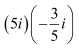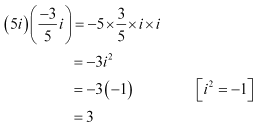Question 2 :

Express the given complex number in the form a + ibi9 + i19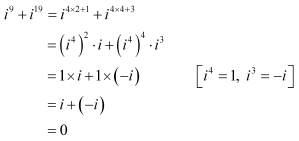Question 3 : Express the given complex number in the form a + ib: i–39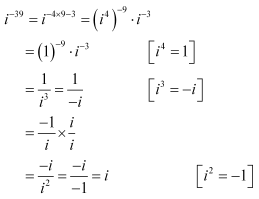Question 4 : Express the given complex number in the form a + ib: 3(7 + i7) + i(7 + i7)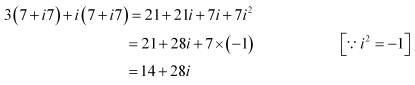Question 5 : Express the given complex number in the form a + ib: (1 – i) – (–1 + i6)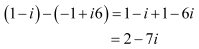Question 6 : Express the given complex number in the form a + ib: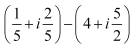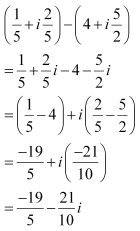Question 7 : Express the given complex number in the form a + ib: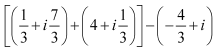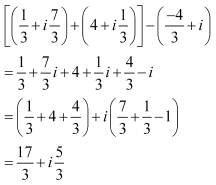Question 8 : Express the given complex number in the form a + ib:  (1 – i)4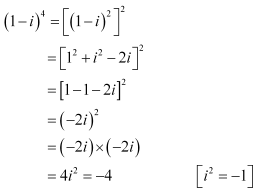Question 9 : Express the given complex number in the form a + ib: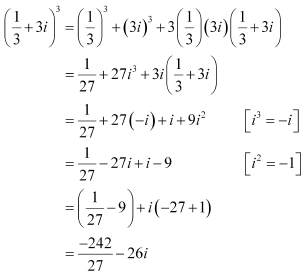Question 10 : Express the given complex number in the form a + ib: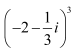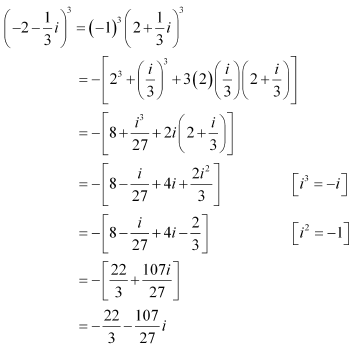Todays Deals### Chapter 5 Complex Numbers and Quadratic Equations Ex-5.1 Contributorskrishan

Name:
Email:

# Latest News# 9000 interview questions in different categories